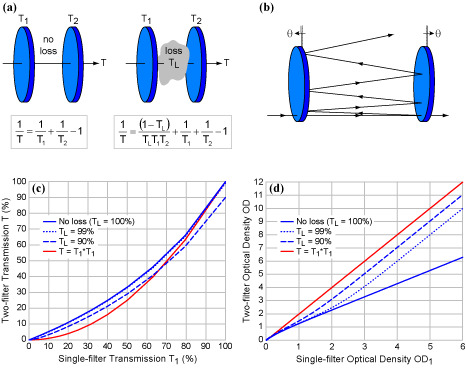Switch Customer
• ##### {{category.Description}}View All Resources

# How to achieve high blocking by combining filters

It is possible to increase blocking by combining two or more filters together.  However, with modern high-performance filters that block light by reflection, one should not try to increase blocking merely by stacking two filters immediately next to one another—combining filters this way only works when multiple reflections between the filters are reduced or eliminated! For example, when two OD 6 filters are combined immediately adjacent to one another, the net OD is actually 6.3, and not OD 12 as one might naïvely assume.  Fortunately, introduction of just a small amount of loss (e.g., by separating and tilting the filters) can quickly increase the net OD to a value close to 12.Figure 1:  (a) When incoherent light is transmitted through two coatings or filters that are immediately adjacent and parallel, the net transmission is not simply the product of the transmission values of the two filters (T ≠ T1 x T2).  Therefore, the net OD is not simply the sum of the individual OD values.  (b) Loss intentionally introduced between the two filters by tilting and separating them makes it possible to make the OD’s approximately add.  (c) Calculated net transmission of incoherent light through two identical, parallel filters as a function of the transmission through one filter, shown on both (c) linear and (d) logarithmic scales.  Note that two OD 6 filters with no loss between them result in a net OD of 6.3, whereas introduction of only 1% (or 10%) loss increases the net OD to 10 (or 11).

As shown in Fig. 1(a), when incoherent (e.g., fluorescence) light is transmitted through two immediately adjacent coatings or filters, the net transmission is not simply the product of the transmission values of the two filters.  Addition of some loss between the two filters decreases the number of multiple reflections, and thus as the loss increases (TL decreases from 100%), the transmission at lower values (higher OD) begins to approach the product of the two transmission values.  Loss might result from a small amount of absorption or scattering on or between the coatings or filters, or it can be intentionally introduced between two filters to increase the net blocking by tilting the filters relative to one another as shown in Fig. 1(b).  Figs. 1(c) and (d) demonstrate the difference between the actual transmission between two adjacent parallel filters (no loss case), compared to the incorrect assumption that the net transmission is the product of individual transmission values (red curve), and the cases of a small amount of loss (1% and 10%) introduced between the two filters.  Note that there is little difference at large values of transmission, but the difference becomes quite dramatic for very small transmission (high OD) values.